Test: Time Varying EMT Fields- 2

# Test: Time Varying EMT Fields- 2 - Electrical Engineering (EE)

Test Description

## 15 Questions MCQ Test Topicwise Question Bank for Electrical Engineering - Test: Time Varying EMT Fields- 2

Test: Time Varying EMT Fields- 2 for Electrical Engineering (EE) 2023 is part of Topicwise Question Bank for Electrical Engineering preparation. The Test: Time Varying EMT Fields- 2 questions and answers have been prepared according to the Electrical Engineering (EE) exam syllabus.The Test: Time Varying EMT Fields- 2 MCQs are made for Electrical Engineering (EE) 2023 Exam. Find important definitions, questions, notes, meanings, examples, exercises, MCQs and online tests for Test: Time Varying EMT Fields- 2 below.
Solutions of Test: Time Varying EMT Fields- 2 questions in English are available as part of our Topicwise Question Bank for Electrical Engineering for Electrical Engineering (EE) & Test: Time Varying EMT Fields- 2 solutions in Hindi for Topicwise Question Bank for Electrical Engineering course. Download more important topics, notes, lectures and mock test series for Electrical Engineering (EE) Exam by signing up for free. Attempt Test: Time Varying EMT Fields- 2 | 15 questions in 45 minutes | Mock test for Electrical Engineering (EE) preparation | Free important questions MCQ to study Topicwise Question Bank for Electrical Engineering for Electrical Engineering (EE) Exam | Download free PDF with solutions
 1 Crore+ students have signed up on EduRev. Have you?
Test: Time Varying EMT Fields- 2 - Question 1

### Consider the following statements: 1. Electric charge at rest produces an electrostatic field. 2. Electric charge in motion produces a magnetic field. 3. Electric and magnetic fields are fundamentally fields of force which originate from electric charges. 4. Faraday’s induction law is given by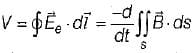Which of the above statements are correct?

Test: Time Varying EMT Fields- 2 - Question 2

### A circular coil begins completely outside any magnetic field, and then during the next 10 sec. it moves completely into a uniform 15T magnetic field. Assuming that the plane of the loop and the direction of motion are both perpendicular to the magnetic field, the magnitude of induced emf in the coil with 25 loops and radius of 7 cm is

Detailed Solution for Test: Time Varying EMT Fields- 2 - Question 2

Since the loop begins outside of any magnetic field, therefore initial magnetic flux is zero.
The final flux is given by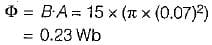Using Faraday’s law, emf induced in the loop is given by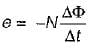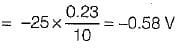∴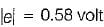Test: Time Varying EMT Fields- 2 - Question 3

### A 18 cm conducting rod attached to a U-shaped conductor moves at a speed of 1 cm/s perpendicular to a magnetic field of strength 37.5T as shown in figure below.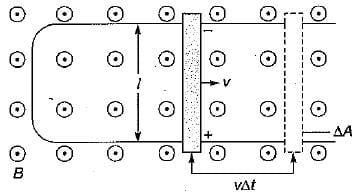What is the emf induced in the conducting rod?

Detailed Solution for Test: Time Varying EMT Fields- 2 - Question 3

The motional emf induced when the conductor’s velocity, length and the magnetic field are all perpendicular is given by
e = BIv
= 37.5 x 0.18 x 0.01
= 0.07 V

Test: Time Varying EMT Fields- 2 - Question 4

What is the strength of the electric field produced in the conductor by the change in flux in the above problem?

Detailed Solution for Test: Time Varying EMT Fields- 2 - Question 4

The electric field produced is given by
E = vB = 0.01 x 37.5
= 0.375 N/C = 0.375 V/m
or, E = 0.38 N/C or V/m

Test: Time Varying EMT Fields- 2 - Question 5

What is the induced emf produced when the radius of one loop or wire in a 12T magnetic field changes from 2 cm to 9 cm in 3 seconds?
Assume that the plane of the loop remains parallel to the direction of the magnetic field.

Detailed Solution for Test: Time Varying EMT Fields- 2 - Question 5

An emf is not induced if the plane of the coil is parallel to the lines of the magnetic field since the magnetic flux through the coil does not change when the coil shrinks.

Test: Time Varying EMT Fields- 2 - Question 6

When a magnetic flux cuts across 200 turns at the rate of 2 Wb/s, the induced voltage is

Detailed Solution for Test: Time Varying EMT Fields- 2 - Question 6

Induced emf,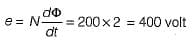Test: Time Varying EMT Fields- 2 - Question 7

A conducting bar can slide freely over two conducting rails as shown in figure.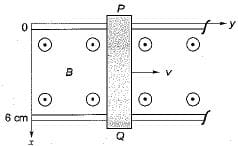What is the magnitude of maximum induced voltage in the bar if the bar is stationed at y = 8 cm and B = 4 cos 106 t azmWb/m2?

Detailed Solution for Test: Time Varying EMT Fields- 2 - Question 7

The transformer emf is given by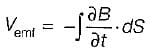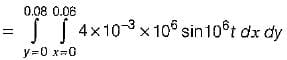= 19.2 sin 106 t V
∴ |Vemf|max = 19.2V

Test: Time Varying EMT Fields- 2 - Question 8

A parallel plate capacitor with plate area of 5 cm2 and plate separation of 3 mm has a voltage 50sin103t applied to its plate. Calculate the displacement current assuming ε = 2ε0.

Detailed Solution for Test: Time Varying EMT Fields- 2 - Question 8(S → Area)
Here,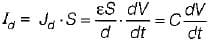Which is the same as the conduction current given by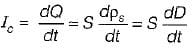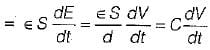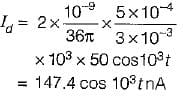Test: Time Varying EMT Fields- 2 - Question 9

The flux through each turn of a 100-turn coil is (t3 - 2t) mWb, where t is in seconds. The induced emf at t = 2 sec. is

Detailed Solution for Test: Time Varying EMT Fields- 2 - Question 9

Given,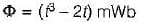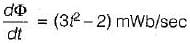Emf induced is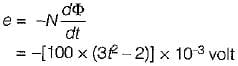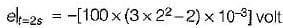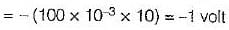Test: Time Varying EMT Fields- 2 - Question 10

A loop is rotating about the y-axis in a magnetic field B = (B0 sinωt) ax mWb/m2. The voltage induced in the loop is due to

Test: Time Varying EMT Fields- 2 - Question 11

The concept of displacement current is a major contribution attributed to

Test: Time Varying EMT Fields- 2 - Question 12

Which of the following expressions does not represent Maxwell’s equations for time-varying fields?

Test: Time Varying EMT Fields- 2 - Question 13

An electromagnetic field is said to be conservative when

Detailed Solution for Test: Time Varying EMT Fields- 2 - Question 13

∇ x E  = 0 for conservative field.

Test: Time Varying EMT Fields- 2 - Question 14

A loop is inside a uniform magnetic field of B = 50 ax mWb/m2 as shown in figure. If side DC of the loop cuts the flux lines at the frequency of 50 Hz and the loop lies in the yz plane at time t = 0, what is the induced emf at t = 5 ms?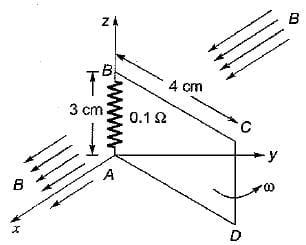Detailed Solution for Test: Time Varying EMT Fields- 2 - Question 14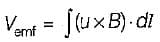Given, dl = dlDC = dZaz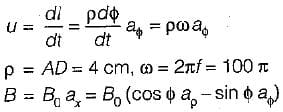and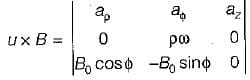= -ρωB0 cos ϕ az
Now, (u x B)·dl = -ρB0ω cos ϕ dz
= - 0.2 π cos ϕ dz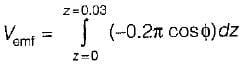= -6π cos ϕ mv
Also, Vemf = - 6π cos (ωt + π/2), at t = 5 ms
∴  |Vemf| = 6 πmV

Test: Time Varying EMT Fields- 2 - Question 15

If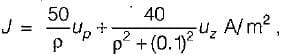the total current crossing the plane z = 0.2 in the uz direction for p < 0.4 is

Detailed Solution for Test: Time Varying EMT Fields- 2 - Question 15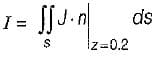or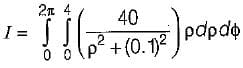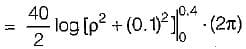or, I = 40π log 17 = 356 A

## Topicwise Question Bank for Electrical Engineering

211 tests
Information about Test: Time Varying EMT Fields- 2 Page
In this test you can find the Exam questions for Test: Time Varying EMT Fields- 2 solved & explained in the simplest way possible. Besides giving Questions and answers for Test: Time Varying EMT Fields- 2, EduRev gives you an ample number of Online tests for practice

211 tests